# Martin Orr's Blog

## Siegel's theorem for curves of genus 0

Posted by Martin Orr on Friday, 28 October 2011 at 12:35

Last time we proved Siegel's theorem on the finiteness of integer points on affine curves of genus at least 1. The theorem applies also to curves of genus 0 with at least 3 points at infinity. I shall give a simple proof that deduces this from the higher genus case, then another proof using Baker's theorem from transcendental number theory which gives an effective bound on the heights of the points.

Theorem. Letbe a number field anda finite set of places of. Letbe an affine-curve of genus 0 such that there are at least 3-points in the projective closure ofwhich are not in. Thenhas finitely many-integer points.

The condition that there should be at least 3 points at infinity is necessary: the affine line is a genus 0 curve with 1 point at infinity and infinitely many integer points, and the curvefor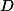a non-square positive integer has 2 points at infinity and infinitely many integer points.

### The unit equation

The unit equation is a special case of Siegel's theorem for genus 0. We give a simple proof that it has finitely many solutions, using Siegel's theorem for higher genus. Then we show that the general Siegel's theorem for any curve of genus 0 can be reduced to the unit equation.

Theorem. Letbe a number field anda finite set of places of. Let.
Then the equationhas finitely many solutions in-units.

Proof 1. The groupis finite (by Dirichlet's unit theorem) so any-units hasfor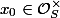andfrom a fixed finite set. Hence there are finitely many equations of the form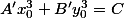which give all the solutions to the unit equation.

But each equationdefines an affine curve of genus 1, so by Siegel's theorem it has finitely many integer solutions.

To see that this is a special case of Siegel's theorem for genus 0, observe that solutions to the unit equation are the same as-integer points on the affine curve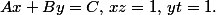Projection onto the-coordinate gives an isomorphism withso this is a genus 0 curve with three points at infinity.

Any genus 0 curve is isomorphic to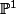minus a finite set of points, so if it has at least 3 points at infinity then it has an injective morphism into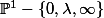for some. This morphism is given by polynomials with coefficients in; by multiplying them by a constant we may suppose that the coefficients are inand so the morphism takes-integral points to-integral points. Then the finiteness of the solutions to the unit equation implies the finiteness of-integer points on the original curve.

### Baker's method

Baker's theorem gives an effective bound on how closely it is possible to approximate zero by a linear combination of logarithms. We can use this to prove the finiteness of solutions to the unit equation, and get an effective bound on their height.

Baker's Theorem. Letbe a number field and. Then there is an effective constant, depending only onand on the completion, such that for any integerswith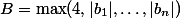, either(This statement of the theorem contains no logarithms, but it is equivalent to a statement thatis either zero or bounded away from zero.)

To apply this to the unit equation, we start with Dirichlet's unit theorem:is isomorphic to the direct product of a finite groupand a free abelian group; letbe a basis for the latter.

Given, define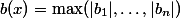. This defines a discrete norm on. We can define another such norm byTo see that the latter is a norm, we need to prove that it is positive for all. This follows from the fact thatfor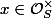. (We need to enlargehere to contain all the archimedean places. Then for an-unit, we havefor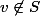and the product formula gives that the sum of the logs of the absolute values is 0.)

Any two discrete norms on a finitely generated free abelian group are equivalent, so there exists a constantsuch that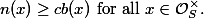Or in other words, for any, there is a valuation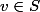for which.

Sinceis finite, it will suffice to prove that for each,has finitely many solutions in-units satisfying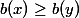and.

So given such a solution, writeand, and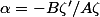.

Then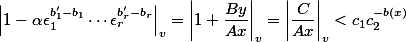for some constants,depending only on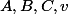.

But by Baker's theorem, the LHS of this inequality is greater than. So we haveandis bounded. The constants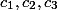are effectively computable, and hence we can get an effective bound for.

Tags maths, number-theory

1. Barinder Banwait said on Sunday, 06 November 2011 at 19:17 :
1. Small typo: In the paragraph before "The Unit Equation", I think you want your curve to be.

2. Why isa norm, that is, why is it non-negative? So far as I can see, all of thecould be negative. Don't we need to boundfrom below as well?

2. Martin Orr said on Monday, 07 November 2011 at 12:36 :

You area right on both counts. Both in the definition ofand in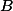in Baker's theorem, thes need to be replaced by.